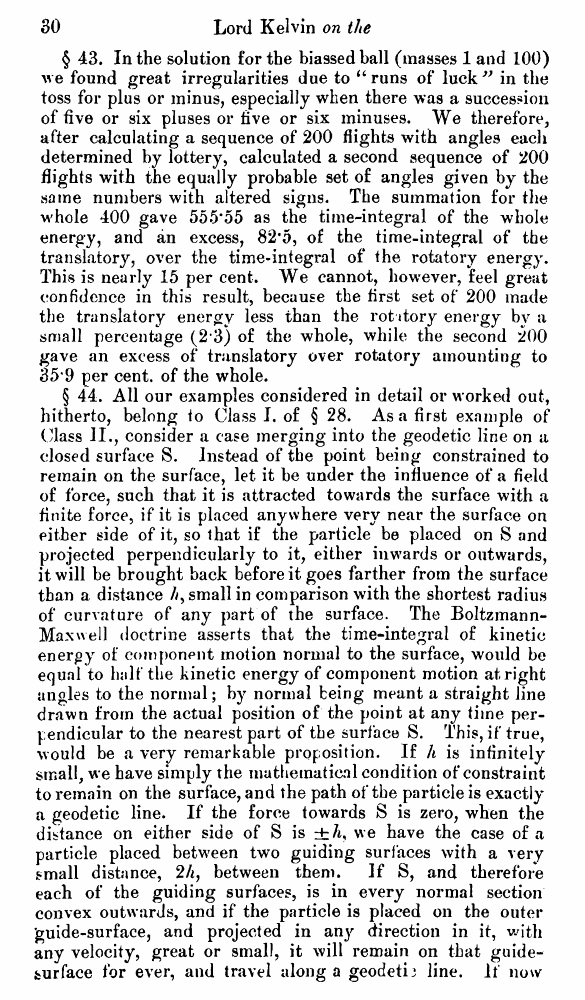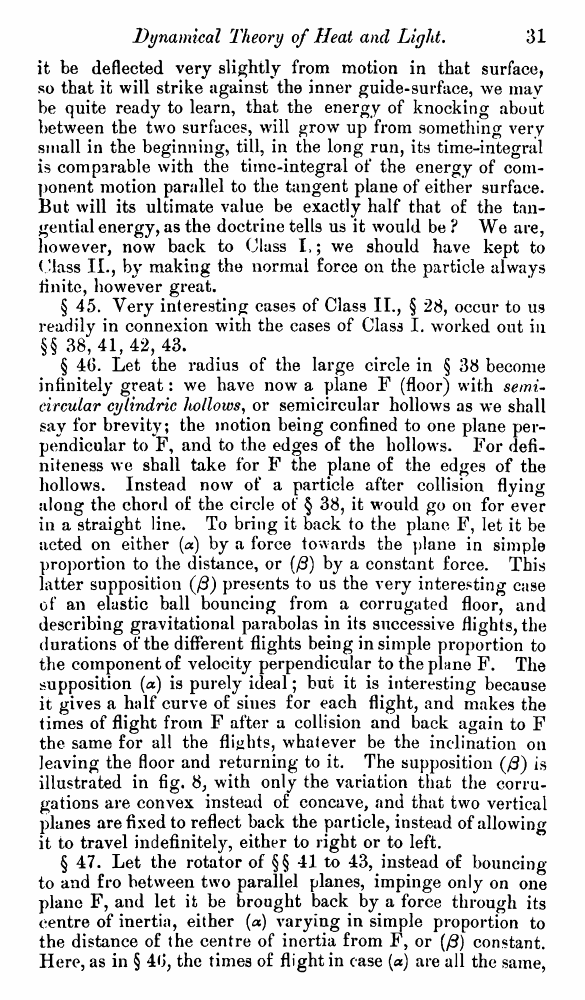# Lord Kelvin. Nineteenth-Century Clouds over the Dynamical Theory of Heat and Light. // Phil. Mag. S. 6. Vol. 2. No. 7. July 1901.

В начало   Другие форматы   <<<     Страница 30   >>>1  2  3  4  5  6  7  8  9  10  11  12  13  14  15  16  17  18  19  20  21  22  23  24  25  26  27  28  29  30 31  32  33  34  35  36  37  38  39  40 § 43. In the solution for the biassed ball (masses 1 and 100) we found great irregularities due to “ runs of luck” in the toss for plus or minus, especially when there was a succession of five or six pluses or five or six minuses. We therefore, after calculating a sequence of 200 flights with angles each determined by lottery, calculated a second sequence of 200 flights with the equally probable set o£ angles given by the same numbers with altered signs. The summation for the whole 400 gave 555*55 as the time-integral of the whole energy, and an excess, 82*5, of the time-integral of the translatory, over the time-integral of the rotatory energy. This is nearly 15 per cent. We cannot, however, feel great confidence in this result, because the first set of 200 made the translatory energy less than the rot itory energy by a small percentage (2 3) of the whole, while the second 200 gave an excess of translatory over rotatory amounting to 35*9 per cent, of the whole. § 44. All our examples considered in detail or worked out, hitherto, belong to Class I. of § 28. Asa first example of ("lass II., consider a case merging into the geodetic line on a closed surface S. Instead of the point being constrained to remain on the surface, let it be under the influence of a field of force, such that it is attracted towards the surface with a finite force, if it is placed anywhere very near the surface on either side of it, so that if the particle be placed on S and projected perpendicularly to it, either inwards or outwards, it will be brought back before it goes farther from the surface than a distance //, small in comparison with the shortest radius of curvature of any part of the surface. The Boltzmann-Maxwell doctrine asserts that the time-integral of kinetic energy of component motion normal to the surface, would be equal to half the kinetic energy of component motion at right angles to the normal; by normal being meant a straight line drawn from the actual position of the point at any time perpendicular to the nearest part of the surface S. This, if true, would be a very remarkable proposition. If h is infinitely small, we have simply the mathematical condition of constraint to remain on the surface, and the path of the particle is exactly a geodetic line. If the force towards S is zero, when the distance on either side of S is we have the case of a particle placed between two guiding surfaces with a very small distance, 2A, between them. If S, and therefore each of the guiding surfaces, is in every normal section convex outwards, and if the particle is placed on the outer 'guide-surface, and projected in any direction in it, with any velocity, great or small, it will remain on that guide-jburface for ever, and travel along a geodetij line. If now it be deflected very slightly from motion in that surface, so that it will strike against the inner guide-surface, we mav be quite ready to learn, that the energy of knocking about between the two surfaces, will grow up from something very small in the beginning, till, in the long run, its time-integral is comparable with the time-integral of the energy of component motion parallel to the tangent plane of either surface. But will its ultimate value be exactly half that of the tangential energy, as the doctrine tells us it would be ? We are, however, now back to Class I,; we should have kept to (.'lass IL, by making the normal force on the particle always Unite, however great. § 45. Very interesting cases of Class II., § 28, occur to us readily in connexion with the cases of Class I. worked out in §§ 38,41,42,43. § 46. Let the radius of the large circle in § 38 become infinitely great: we have now a plane F (floor) with semicircular cylindric hollows, or semicircular hollows as we shall say for brevity; the motion being confined to one plane perpendicular to F, and to the edges of the hollows. For definiteness we shall take for F the plane of the edges of the hollows. Instead now of a particle after collision flying along the chord of the circle of § 38, it would go on for ever in a straight line. To bring it back to the plane F, let it be acted on either (a) by a force towards the plane in simple proportion to the distance, or (/3) by a constant force. This latter supposition (/3) presents to us the very interesting case of an elastic ball bouncing from a corrugated floor, and describing gravitational parabolas in its successive flights, the durations of the different flights being in simple proportion to the component of velocity perpendicular to the plane F. The supposition (a) is purely ideal; but it is interesting because it gives a half curve of sines for each flight, and makes the times of flight from F after a collision and back again to F the same for all the flights, whatever be the inclination on leaving the floor and returning to it. The supposition (/3) is illustrated in fig. 8; with only the variation that the corrugations are convex instead of concave, and that two vertical planes are fixed to reflect back the particle, instead of allowing it to travel indefinitely, either to right or to left. § 47. Let the rotator of §§ 41 to 43, instead of bouncing to and fro between two parallel planes, impinge only on one plane F, and let it be brought back by a force through its centre of inertia, either (a) varying in simple proportion to the distance of the centre of inertia from F, or (/?) constant. Here, as in § 40, the times of flight in case (a) are all the same,